### Home > MC1 > Chapter 4 > Lesson 4.1.4 > Problem4-46

4-46.
1. . Kenali wants to cut a piece of rope into three equal pieces, and she wants to have a 10-foot piece remaining. Help her figure out how long to make each of the three equal pieces for each total length of rope given below. Homework Help ✎

1. A 25-foot piece of rope (find m).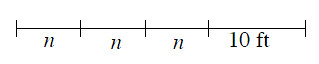2. A 310-foot piece (find x).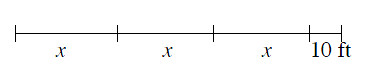3. A 13-foot piece (find j).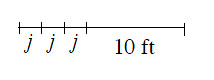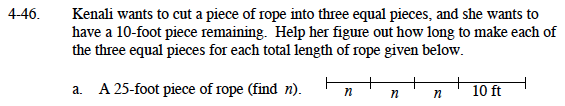First, subtract 10 from 25 to find the amount left for the three equal parts.

25 feet − 10 feet = 15 feet

n = 5 feet

Next, determine how long each of the three pieces will be. If three pieces must add together to be 15 feet, how long are they?

$\frac{15}{3}=5\text{ feet}$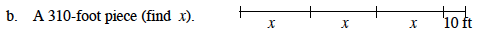The process for solving this problem is the same as in part (a).
Remember to subtract 10 feet from the total before dividing it into three parts.

x = 100 feet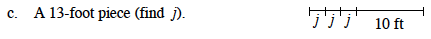Follow the steps outlined in part (a).